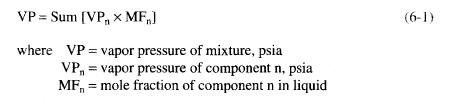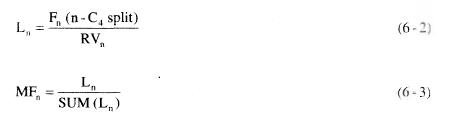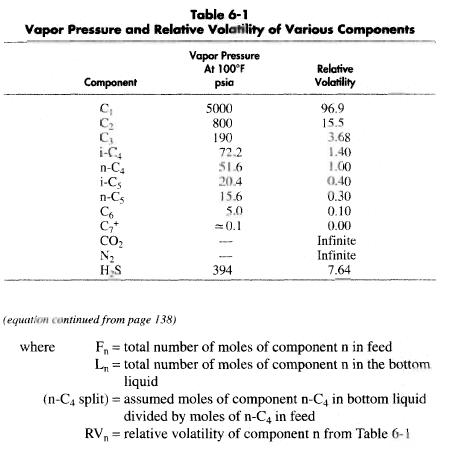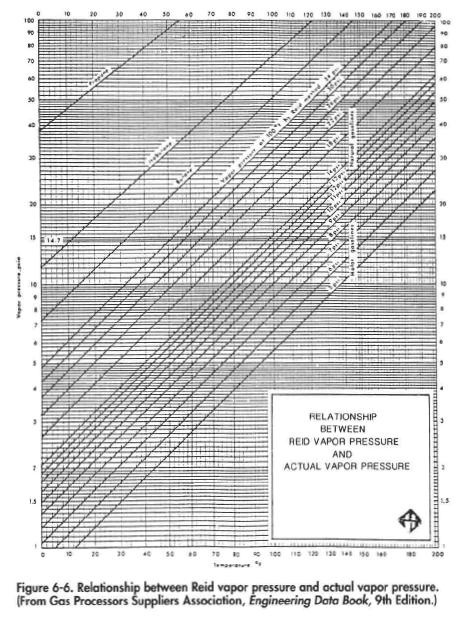﻿ Condensate Stabilizer Design | Oil & Gas Process Engineering

## Condensate Stabilizer Design

It can be seen from the previous description that the design of both a cold-feed stabilizer and a stabilizer with reflux is a rather complex and involved procedure. Distillation computer simulations are available that can be used to optimize the design of any stabilizer if the properties of the feed stream and desired vapor pressure of the bottoms product are known. Cases should be run of both a cold-feed stabilizer and one with reflux before a selection is made. Because of the large number of calculations required, it is not advisable to use hand calculation techniques to design a distillation process. There is too much opportunity for computational error.

Normally, the crude or condensate sales contract will specify a maximum Reid Vapor Pressure (RVP). This pressure is measured according to a specific ASTM testing procedure. A sample is placed in an evacuated container such that the ratio of the vapor volume to the liquid volume is 4 to 1. The sample is then immersed in a 100°F liquid bath. The absolute pressure then measured is the RVP of the mixture.

Since a portion of the liquid was vaporized to the vapor space, the liquid will have lost some of its lighter components. This effectively changes the composition of the liquid and yields a slightly lower vapor
pressure than the true vapor pressure of the liquid at 100°F. Figure 6-6 can be used to estimate true vapor pressure at any temperature from a known RVP.

The inherent error between true vapor pressure and RVP means that a stabilizer designed to produce a bottoms liquid with a true vapor pressure equal to the specified RVP will be conservatively designed. The vapor pressures of various hydrocarbon components at 100°F are given in Table 6-1.

The bottoms temperature of the tower can be approximated if the desired vapor pressure of the liquid is known. The vapor pressure of a mixture is given by:To estimate the desired composition of the bottom liquid, the vapor pressures of the different components at 100°F can be assumed to be a measure of the volatility of the component. Thus, if a split of n~C4 is assumed, the mole fraction of each component in the liquid can be estimated from:To determine the compositon of the bottom liquid, assume a split of n-C4 and compute MFn from Equations 6-2 and 6-3. The vapor pressure can then be computed from Equation 6-1. If the vapor pressure is higher than the desired RVP choose a lower number for the n-C4 split. If the calculated
vapor pressure is lower than the desired RVP, choose a higher number for the n~C4 split. Iterate until the calculated vapor pressure equals the desired RVP.

The bottoms temperature can then be determined by calculating the bubble point of the liquid described by the previous iteration at the chosen operating pressure in the tower. This is done by choosing a temperature, determining equilibrium constants from Chapter 3, Volume I , and computing:

If C is greater than 1,0, the assumed temperature is too high. If C is lower than 1.0, the assumed temperature is too low. By iteration a temperature can be determined where C = 1.0.

Typically, bottoms temperatures will range from 200~4QO°F depending on operating pressure, bottoms composition, and vapor pressure requirements. Temperatures should be kept to a minimum to decrease the heat requirements, limit salt build-up, and prevent corrosion problems.

When stabilizer operating pressures are kept below 200 psig the reboiler temperatures will normally be below 300°R A water glycol heating medium can then be used to provide the heat. Higher stabilizer operating pressures require the use of steam- or hydrocarbon-based heating mediums. Operating at higher pressures, however, decreases the flashing of the feed on entering the column, which decreases the amount of feed cooling required. In general, a crude stabilizer should be designed to operate between 100 and 200 psig.